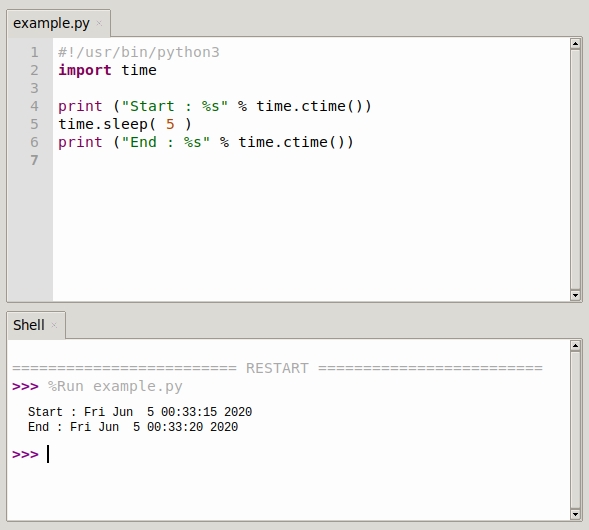# Python time.sleep()

The sleep() method executes the program after pausing for a given number of seconds. This parameter can be a floating-point number to represent a more accurate sleep time.

The actual abort time may not be as long as requested, as any captured signal will terminate the program that sleep() next executes that signal capture.

## syntax

The following is the syntax of the sleep() method.

``time.sleep(t)``

Parameter t - This is the number of seconds to pause the execution. return value

This method does not return any values.

## time.sleep() example

The following example illustrates the use of the sleep() method.

``````#!/usr/bin/python3
import time

print ("Start : %s" % time.ctime())
time.sleep( 5 )
print ("End : %s" % time.ctime())``````

When we run the above program, it produces the following results.

``````Start : Mon Feb 17 12:04:42 2017
End : Mon Feb 17 12:04:47 2017``````

### example 2

The sleep function in Python can pass decimals in, and then it’s ready for a millisecond delay

``````# Example: loop for 1 second
import time
i = 1
while i = 3:
print(i) # Output i
i += 1
time.sleep(1) # Sleep for 1 second

# Example: cycle every 100 milliseconds
import time
i = 1
while i = 3:
print(i) # Output i
i += 1
time.sleep(0.1) # Sleep for 0.1 seconds``````### c sleep

You can use python’s time module to implement a sleep function similar to the one in C

The code is as follows.

``````import time
def sleep(mytime=''):
time.sleep(mytime)

print('call sleep')
sleep(5)#sleep 5s

print('sleep end')``````